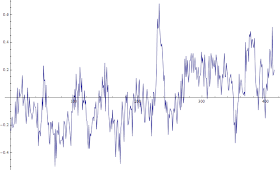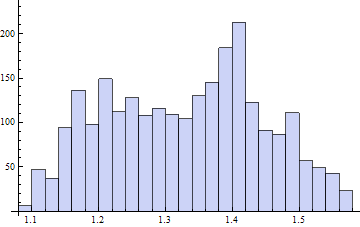## Wednesday, June 05, 2013

### Sea temperature trend: 1.35 ± 0.15 °C per century

I made the following easy-to-understand calculation of the warming trend, including an error margin, of the global sea surface temperatures since the late 1970s, as seen by the UAH AMSU satellite dataset.First, I loaded the file of the monthly data, isolated the third temperature-like column, the global ocean temperature anomaly, and calculated the linear regressions.

It's straightforward to use one simple Mathematica command to compute the slope of the linear regression but the non-trivial addition I made was an estimate of the error margin of the resulting slope. My logic is that for different initial months and final months of the interval, you get different slopes. Then you draw the histogram and the width of this histogram approximately informs you about the error margin of the slope.

So I picked the interval from the $$i$$-th month of the dataset through the $$j$$-th month from the end of the dataset and allowed $$i,j$$ to be integers between one and fifty. One gets 2,500 different slopes from the linear regression. When the month-on-month average slope is multiplied by 1,200 to get the warming per century, those 2,500 slopes are distributed along the following histogram:One may easily compute the mean value 1.33 °C per century while the root-mean-square width of the curve is 0.12 °C.$\ddfrac{T}{t}\sim (1.33\pm 0.12)^\circ {\rm C} / {\rm century}$ It's also possible to replace the number 50 by another number of months, like 60, and the qualitative conclusions are unchanged.

This really means that the data from the last 33 years – when the observed warming trend was faster than in longer intervals or previous intervals, so we're likely to get an overestimate – the warming trend was just 1.35 °C per century with a relatively small error margin. In particular, we can't exclude that the "right" warming trend is below 1 °C per century. However, we can rather reliably exclude the hypothesis that the centennial trend exceeds 2 °C per century.

If you need some very simple Mathematica code I used:
a = Import["http://vortex.nsstc.uah.edu/data/msu/t2lt/uahncdc.lt",
"Table"];
aaa = a[[2 ;; -12]][[All, 3]];
more = a[[2 ;; -12]][[All, 5]];
laaa = Length[aaa]

ListLinePlot[more]

trends = {};
For[i = 1, i <= 50, i++,
For[j = 1, j <= 50, j++,
morekus = more[[i ;; -j]];
trend = D[Normal[LinearModelFit[morekus, x, x]], x]*1200;
trends = trends~Join~{trend};
]
]

Histogram[trends]
avtrend = Total[trends]/2500
Sqrt[Total[trends^2 - avtrend^2]/2500]
I chose the sea temperatures because they seem to be less variable in the short run; the nearly white noise apparently contributing to the temperatures has a smaller prefactor. The latest, May 2013 temperature anomaly which sits at –0.01 °C, wasn't incorporated to the calculation yet. It would reduce the trends but just by a very tiny amount.

Needless to say, nothing guarantees that the underlying trend implicitly assumed above to be linear will remain constant in the future. Climatologists are often naively describing the temperatures as a combination of a superfast, nearly white noise (very high frequencies of the randomness) and a superslow, nearly linear and permanent, increase of the temperature (very low frequencies). In reality, there are contributions from many characteristic intermediate timescales, including one or two years or so from El Niño cycles, decades from PDO and AMO and all these things, and probably many other sub-centennial and near-centennial and multi-centennial cycles, some of which are more regular or periodic or predictable than others while others are chaotic.

Even the assumption that the cherry-picked high trend is going to continue doesn't look worrisome in any sense and the underlying trends are safely lower than the lower end point of the IPCC interval.

Note that the IPCC should publish the fifth report, AR5, later in 2013. I am not too curious what will happen but I am still approximately infinitesimally curious how the usual talking points by these mostly dishonest hired guns will change or not change relatively to AR4. ;-)

1.It seems to me that the air is gradually leaking out of the global warming hysteria. I wonder how long before the first postmortem is published in the mainstream press. I'd guess in about five years.

2.Since when is a step function shortly after the big spike a Trend?
What is the trend before the spike and what is the trend after step up?

3.Brian G ValentineJun 5, 2013, 9:13:00 PM

Nobody mentions geothermal heat as an apparent source of warming in the Northern hemisphere; one cannot assume the geothermal gradient to be constant any more than one can assume that tectonic plates are static.

I think this is the primary source of any "warming" to be found in the absense of solar variation.

4.Brian G ValentineJun 5, 2013, 9:18:00 PM

Sorry to be skeptical, but the known repeated use of Ponzi schemes made few less gullible.

5.Lubo, you're presenting this data as "sea temperature" and "the global ocean temperature anomaly". It isn't. It's lower troposphere temperature anomalies over the ocean.

Regards

6.Would the results vary much if you filtered in the frequency domain?